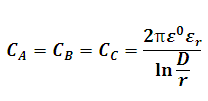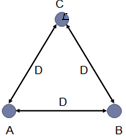# Capacitance of Symmetric Three Phase Transmission Line Calculator

This Calctown Calculator calculates the capacitance of a symmetrically spaced three phase transmission line.

Here e0 = 8.854 * 10-12. Please enter er accordingly.

unit
m
m

#### Result

FClick here to view image

where

ε0 = Dielectric constant in vaccum

εr = Dielectric ratio

D = distance between the lines

r = radius of conductors

Ca, Cb, Cc are Capacitances of phase a, b and c respectively